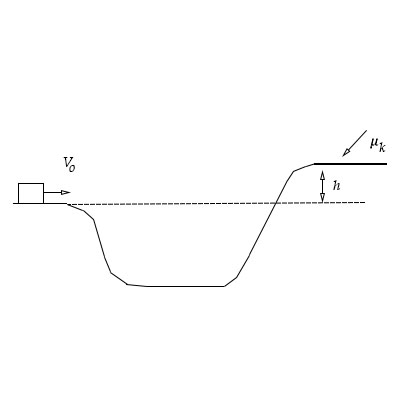# A small block slides along a track from one level to a higher level, by moving through an...

## Question:

A small block slides along a track from one level to a higher level, by moving through an intermediate valley. The track is frictionless until the block reaches the higher level. There a frictional force stops the block in a distance {eq}d {/eq}. Assume that the block's initial speed is {eq}10 \ m/s {/eq}, the height difference {eq}h {/eq} is {eq}3.3 \ m {/eq}, and {eq}\mu_k {/eq} is {eq}0.87 {/eq}. Find the distance {eq}d {/eq} that the block travels on the higher level before stopping.## Work Done:

The force has the tendency to change the state of inertia of the body. If the body is displaced in the applied force direction, then the work done on the body is equal to the product of the applied force and the displacement.

Given data

• The value of the initial speed is {eq}u = 10\;{\rm{m/s}} {/eq}
• The value of the height difference is {eq}h = 3.3\;{\rm{m}} {/eq}
• The value of the coefficient of kinetic friction is {eq}{\mu _k} = 0.87 {/eq}

The expression for the kinetic energy is,

{eq}K.E = \dfrac{1}{2}m{u^2} {/eq}

The expression for the potential energy is,

{eq}P.E = mgh {/eq}

The expression for the work done considering the friction is,

{eq}W = {\mu _k}mgd {/eq}

The expression for the conservation of energy is,

{eq}\begin{align*} K.E &= P.E + W\\ \dfrac{1}{2}m{u^2} &= mgh + {\mu _k}mgd\\ \dfrac{1}{2}{u^2} &= gh + {\mu _k}gd \end{align*} {/eq}

Substitute the values in the above equation, with {eq}g = 9.81 \text{ m/s}^2{/eq}:

{eq}\begin{align*} \dfrac{1}{2}{\left( {10} \right)^2} &= 9.81\left( {3.3} \right) + \left( {0.87} \right)9.81\left( d \right)\\ d &= 2.1\;{\rm{m}} \end{align*} {/eq}

Thus, the distance traveled on the higher level is {eq}2.1\;{\rm{m}} {/eq}.